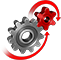# WolframSystemModeler

## PI-controlled Tank System

A classical control problem is to control the water level in a series of tanks. Here, we explore the workings of three PI controllers used in a three-tank system. The tank model contains dynamic diagrams, which is a graphical feature that can be built into any model and provides a fast and automatic animation of the system.

### Visualization Using Dynamic Diagrams

Dynamic diagrams is a dynamic graphical feature that can be built into SystemModeler models. Such diagrams can then be animated in Mathematica, providing an easy-to-use way of visualizing SystemModeler models.The tank system with an input of liquid from "liquidSource". The source initially provides a constant input and then triples it after a certain amount of time. The PI controllers receive a liquid height indication from their respective tanks and then return a process control signal that is based on that indication.
Follow the process as the liquid flows from the source into the three tanks, and see how the tank levels are regulated by the PI controllers.

Dynamic Diagrams

Visualize the dynamics of a PI-regulated tank system with built-in dynamic diagrams.

## Wolfram System Modeler

Questions? Comments? Contact a Wolfram expert »